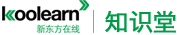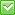<%/*%> <%*/%>热门：已解决问题
math

<div style="margin: 0in 0in 0pt">3-Q2
On saturday morning, Malachi will begin a camping vacation and he will return home at the end of the first day on which it rains. If on the first three days of the vacation the probability of rain on each day is 0.2, what is the probability that Malachi will return home at the end of the day on the following Monday?

A. 0.008   B.0.128   C.0.488   D.0.512   E.0.640

3-Q6
If x and k are integers and (12^x)(4^2x+1)=(2^k)(3^2), what is the value of k?
A.5   B.7   C.10   D.12   E.14

3-Q14
<div style="margin: 0in 0in 0pt">How many seconds will it take for a car that is traveling at a constant rate of 45 miles per hour to travel a distance of 22 yards? (1 mile=1160 yards)
A.8   B.9   C.10   D.11   E.12
</div></div>最佳答案

The 3 day vacation would start on Saturday. hence the 3 days will be Saturday, Sunday and Monday.
We need to find the probability of raining on Monday. This means that it did not rain on first 2 days.
Since probablility of rain is 0.2, hence probability of not raining is 1-0.2 = 0.8
Hence we have the following:

DAY Saturday Sunday Monday
Probability 0.8 0.8 0.2

Hence the required probability is 0.8*0.8*.2 = 0.128

1 mile=1160 yards)是错误的，应该是1 mile=1760 yards)